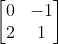## Inverse Matrix

What is the inverse of the shown matrix?Expand Hint
The inverse of a square $$n\times n$$ matrix is:
$$A^{-1}=\frac{adj(A)}{|A|}$$$where $$|A|$$ is the determinant of matrix A, and $$adj(A)$$ is the adjoint of matrix A, which is obtained by replacing $$A^T$$ elements with their cofactors. Hint 2 $$\begin{bmatrix}a & b\\ c & d\end{bmatrix}^{-1}=\frac{1}{ad-bc}\begin{bmatrix}d & -b\\ -c & a\end{bmatrix}$$$
The inverse of a square $$n\times n$$ matrix is:
$$A^{-1}=\frac{adj(A)}{|A|}$$$where $$|A|$$ is the determinant of matrix A, and $$adj(A)$$ is the adjoint of matrix A, which is obtained by replacing $$A^T$$ elements with their cofactors. Thus, for a 2 x 2 matrix: $$\begin{bmatrix}a & b\\ c & d\end{bmatrix}^{-1}=\frac{1}{ad-bc}\begin{bmatrix}d & -b\\ -c & a\end{bmatrix}$$$
Therefore,
$$\begin{bmatrix}0 & -1\\ 2 & 1\end{bmatrix}^{-1}=\frac{1}{(0)(1)-(-1)(2)}\begin{bmatrix}1 & -(-1)\\ -2 & 0\end{bmatrix}$$$$$=\frac{1}{0+2}\begin{bmatrix}1 & 1\\ -2 & 0\end{bmatrix}=\frac{1}{2}\begin{bmatrix}1 & 1\\ -2 & 0\end{bmatrix}=\begin{bmatrix}0.5 & 0.5\\ -1 & 0\end{bmatrix}$$$
The solution can be verified by multiplying the original matrix with its inverse. The resultant is the identity matrix ( $$n\times n$$ matrix with 1’s on the diagonal and 0’s everywhere else).
$$[A][A]^{-1}=[A]^{-1}[A]=[I]$$$where $$[I]$$ is the identity matrix, $$[A]$$ is the original matrix, and $$[A]^{-1}$$ is the inverse matrix. $$\begin{bmatrix}0.5 & 0.5\\ -1 & 0\end{bmatrix}$$$
Similar Problems from FE Sub Section: Inverse

Similar Problems from FE Section: Matrices